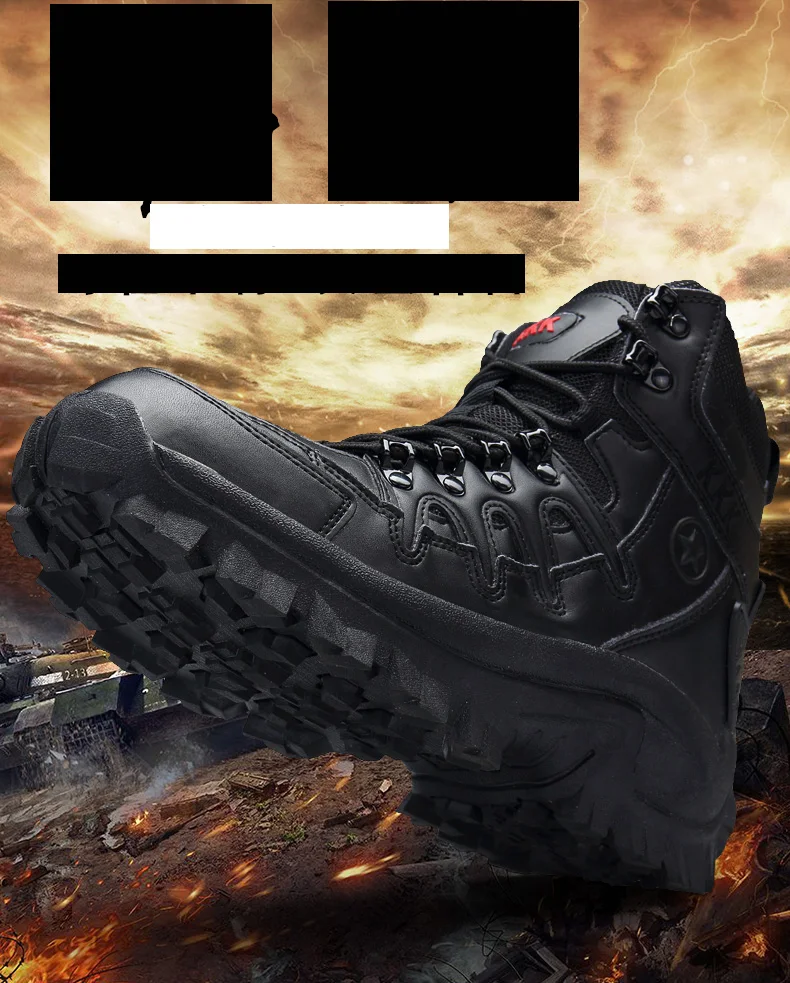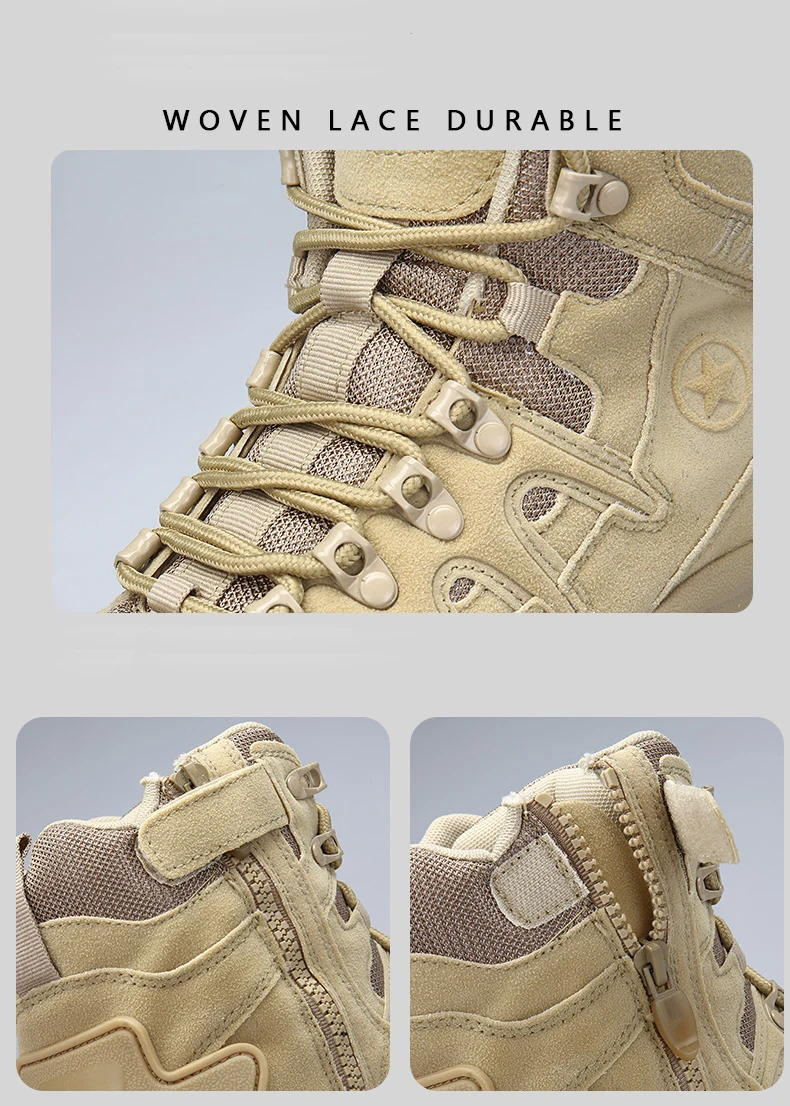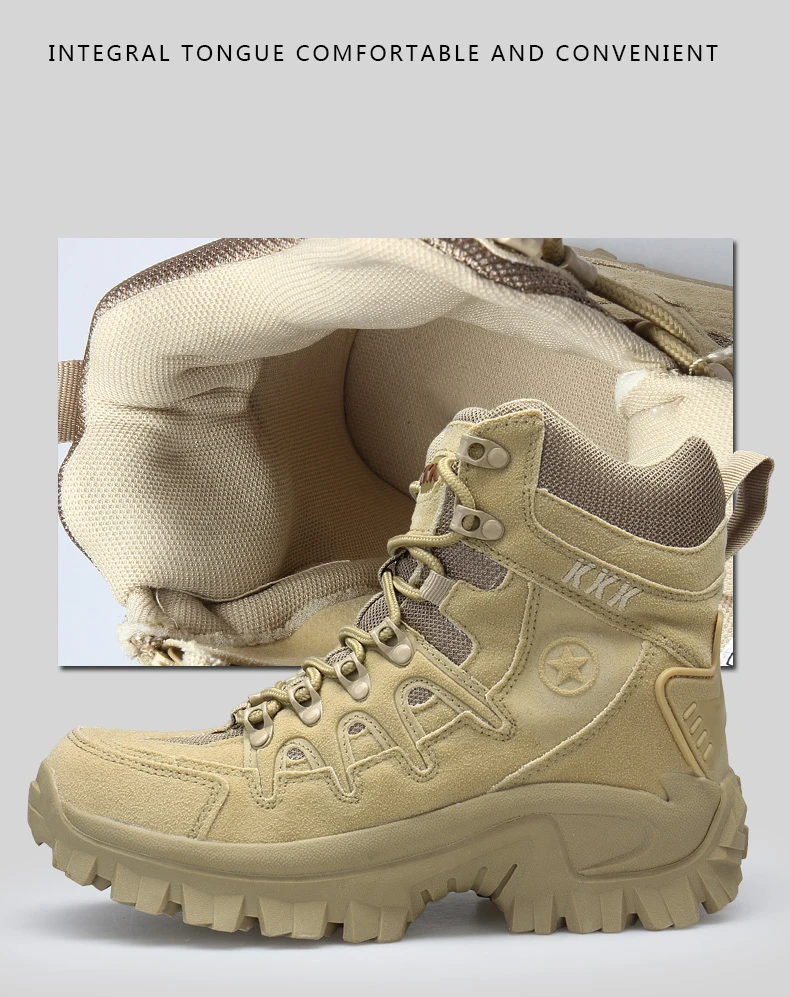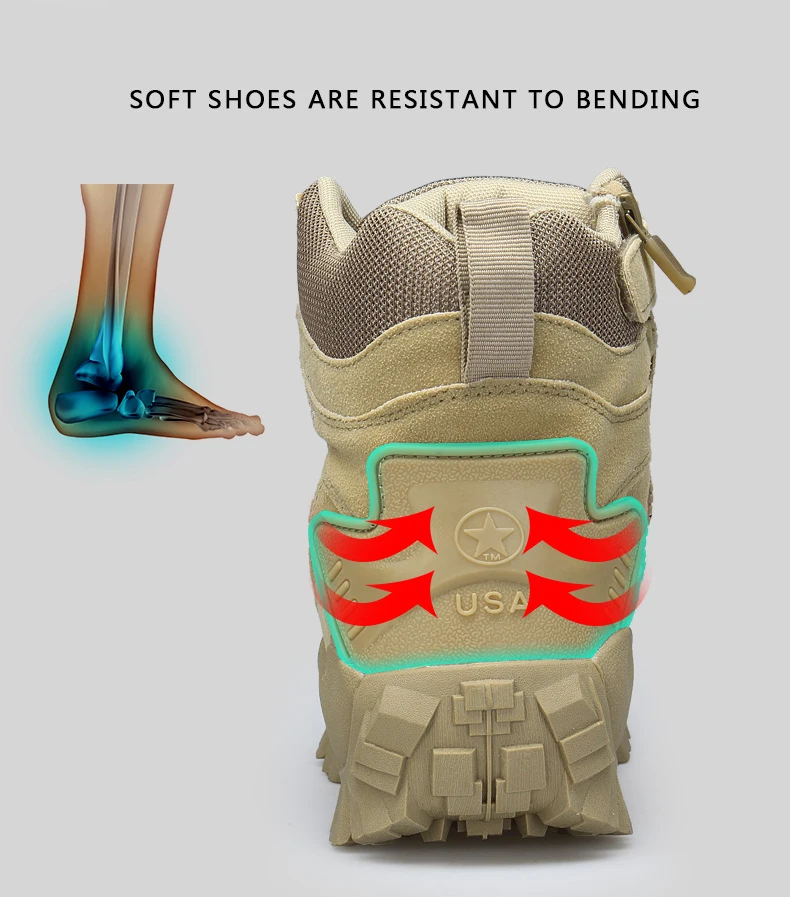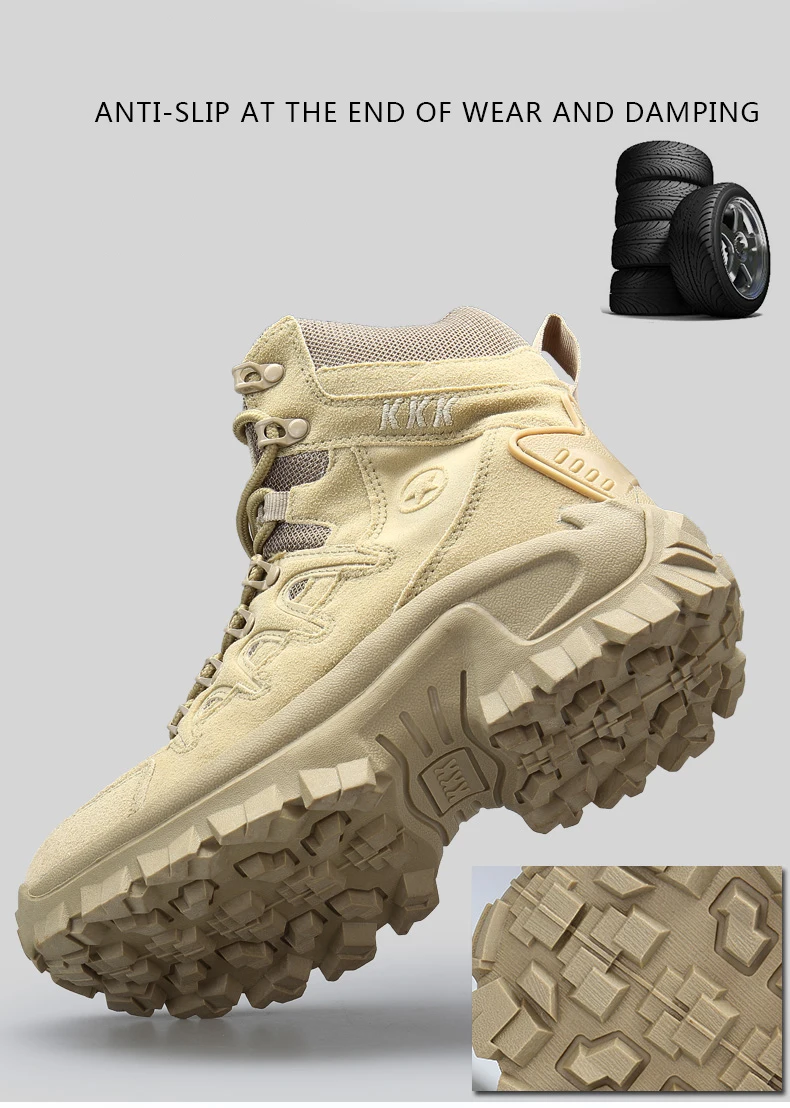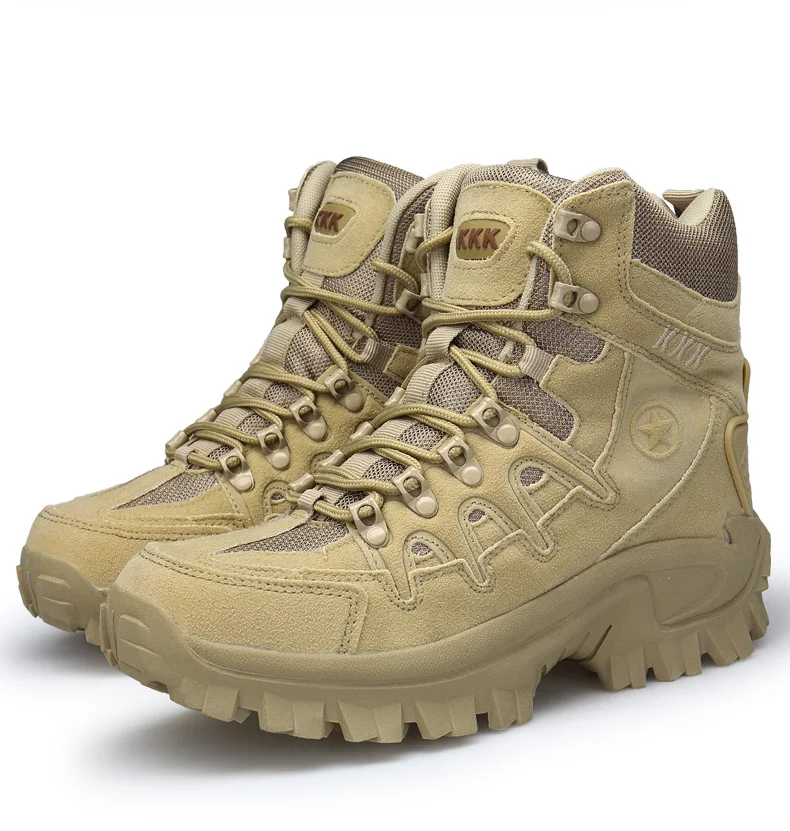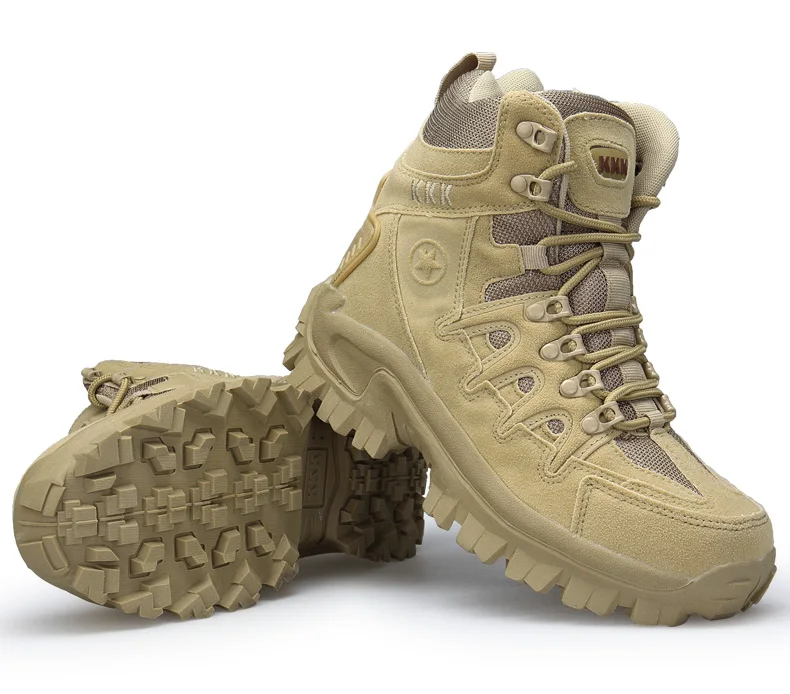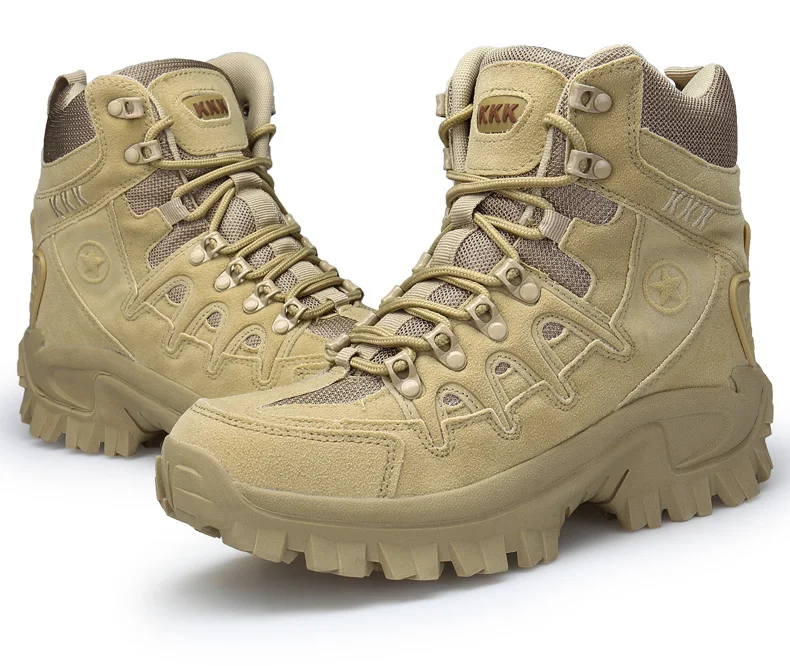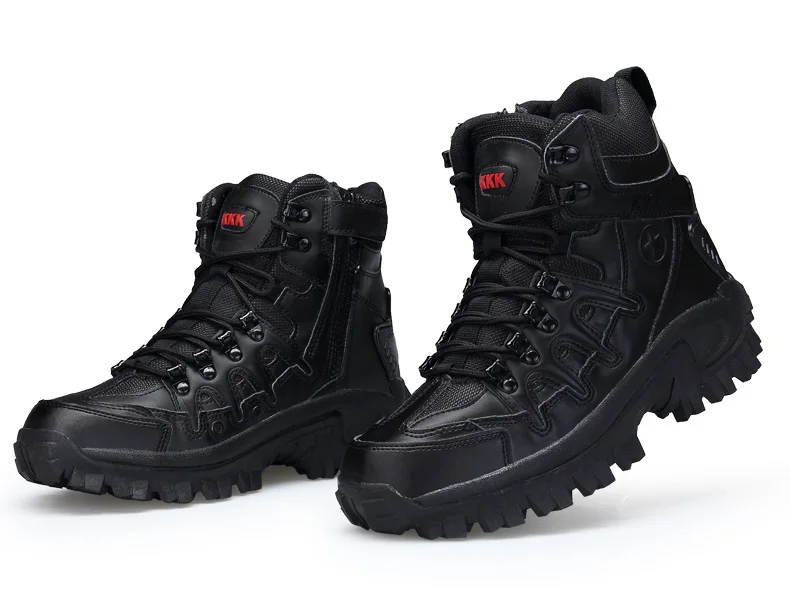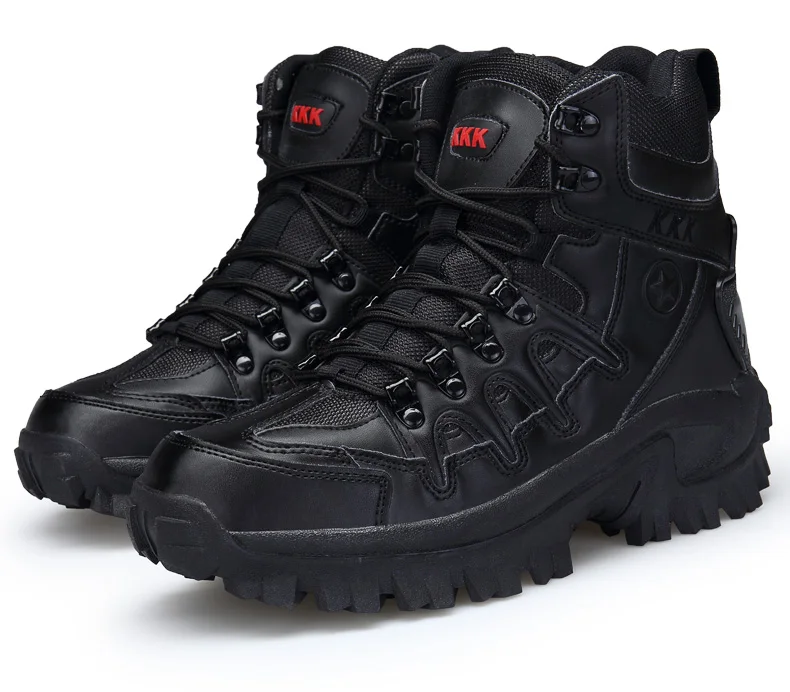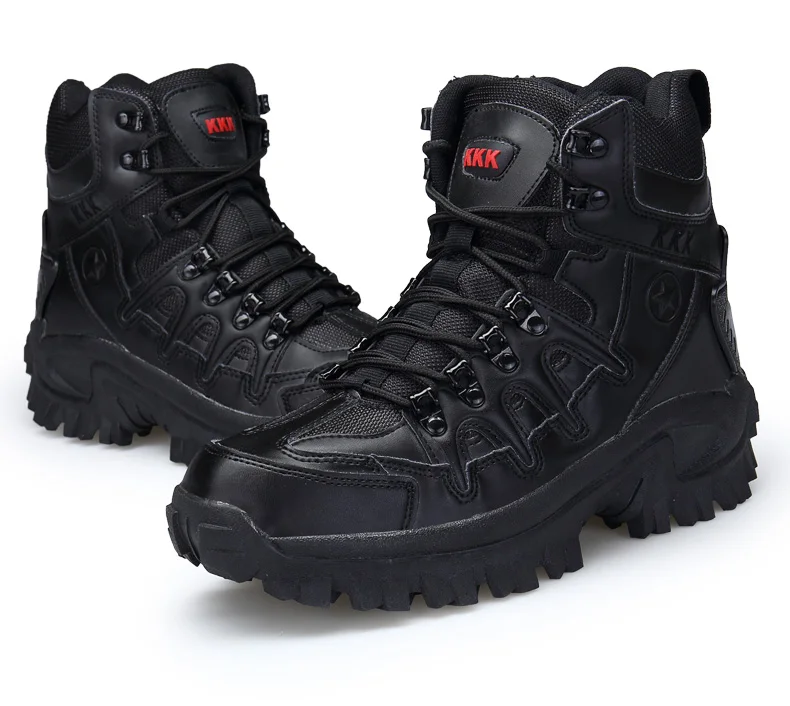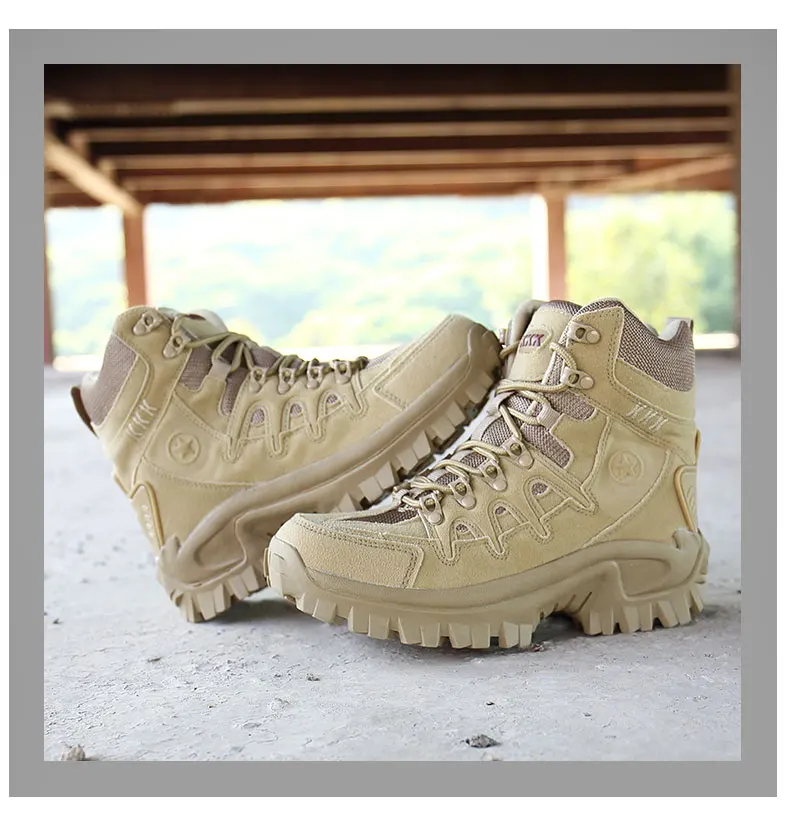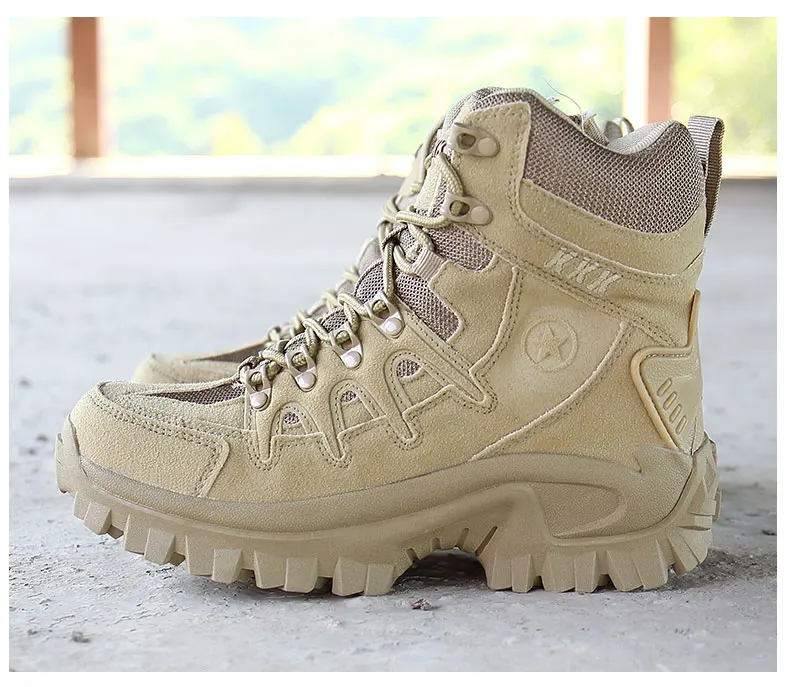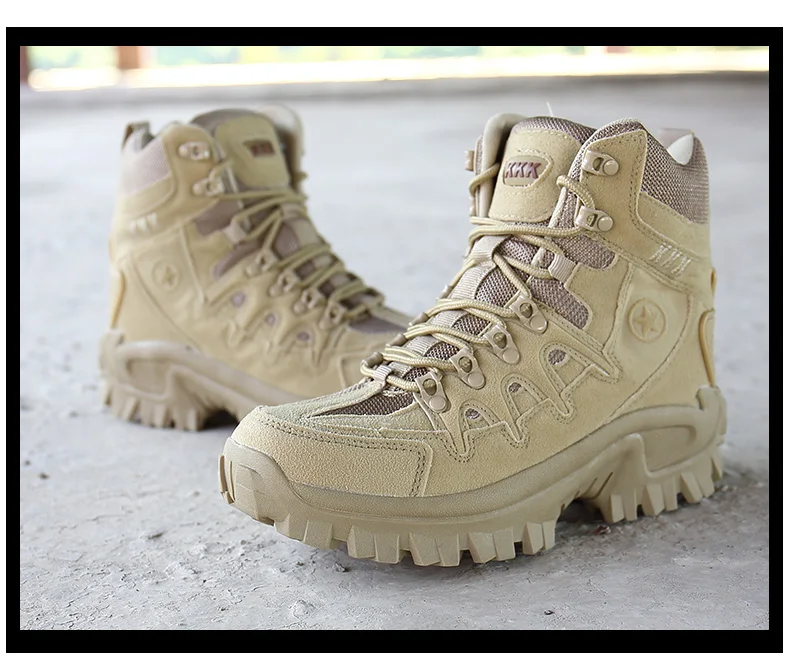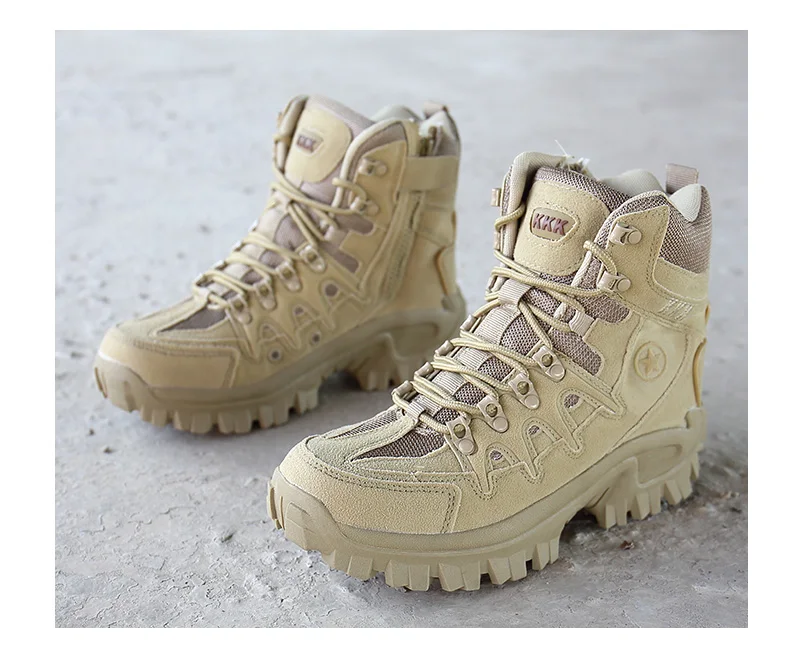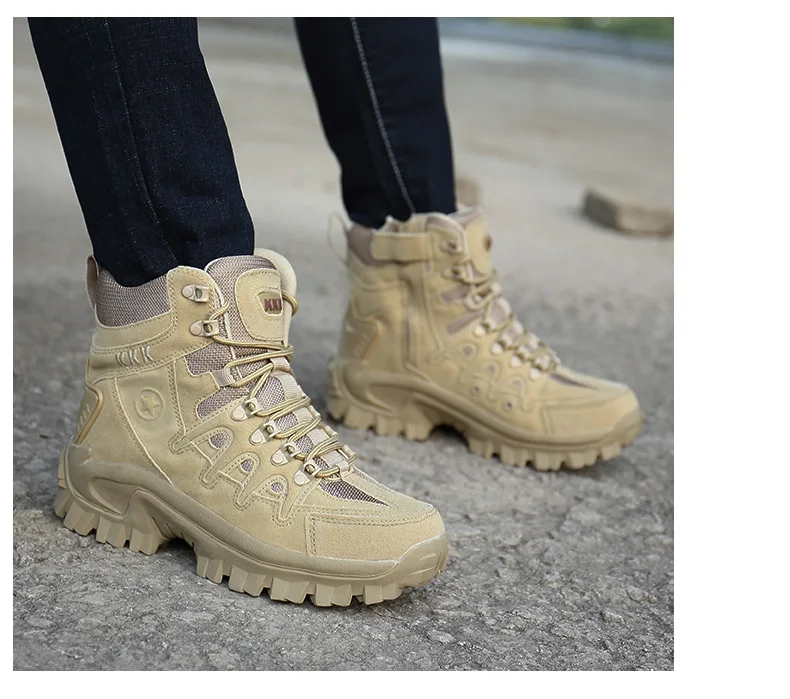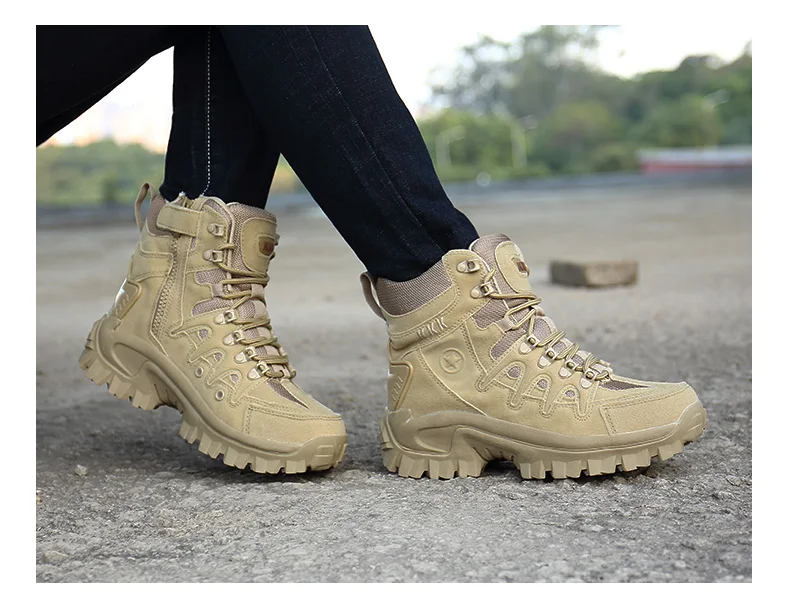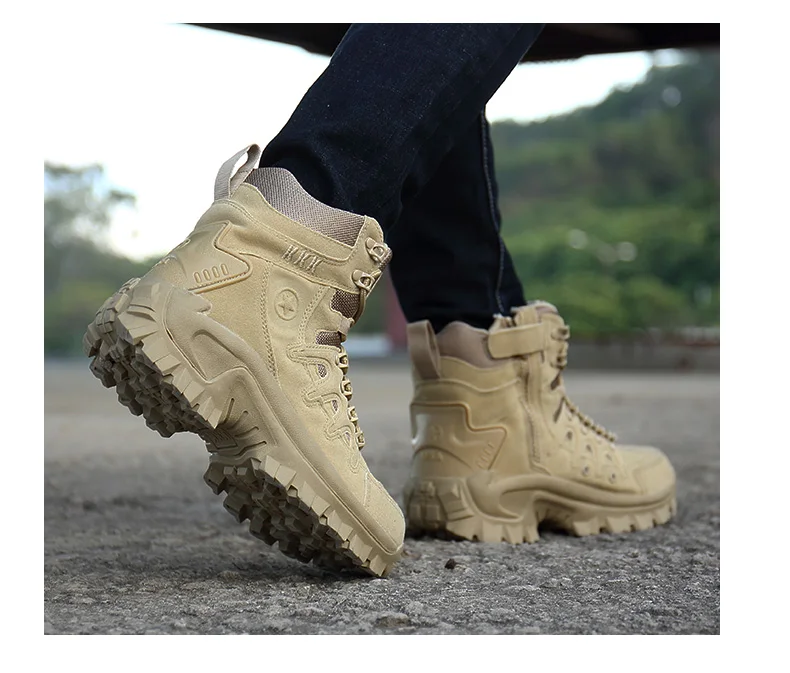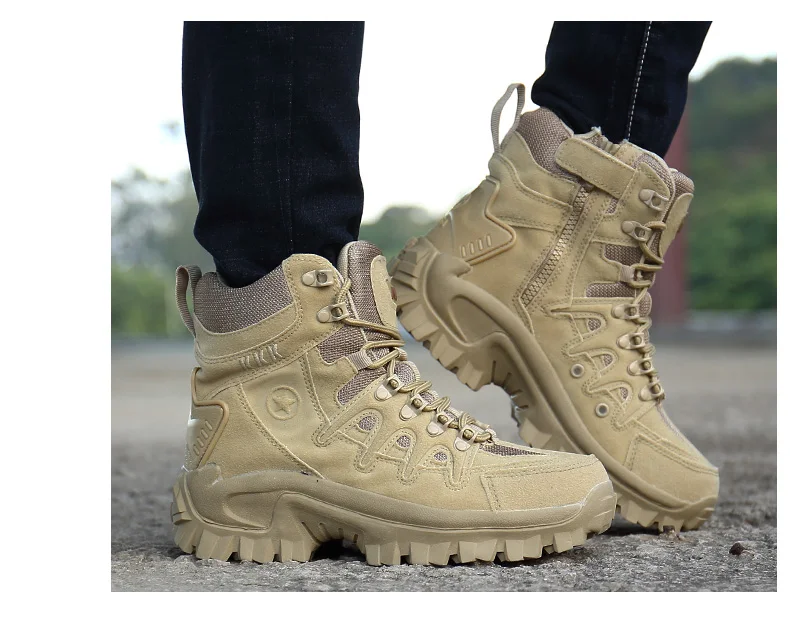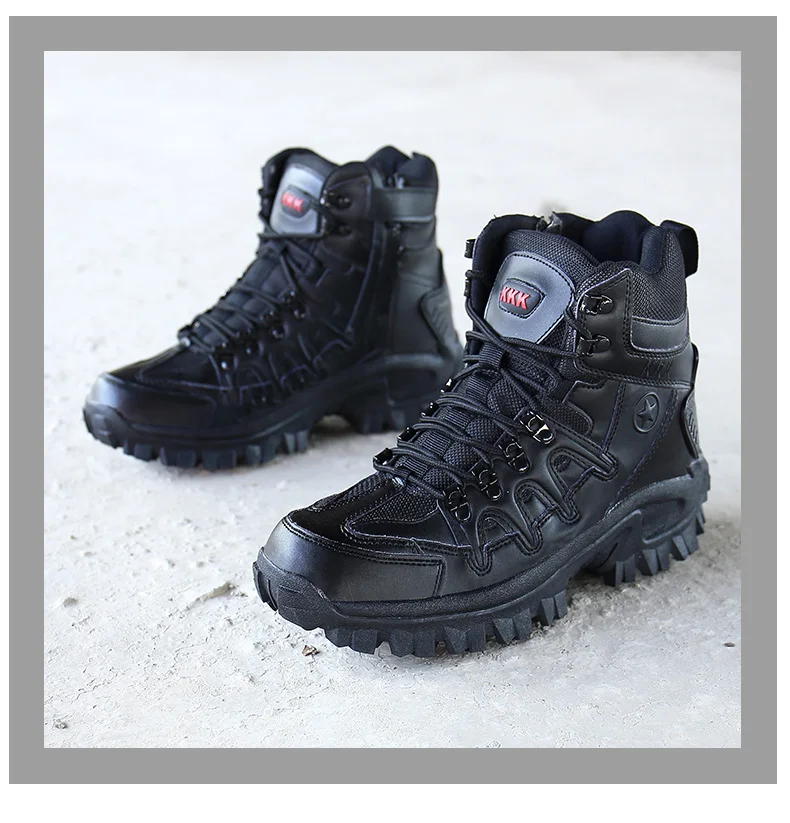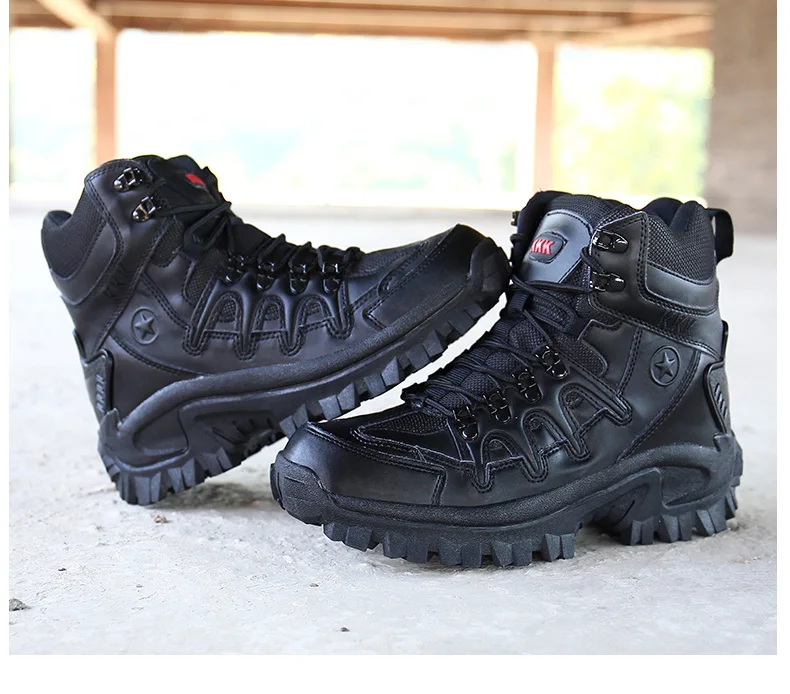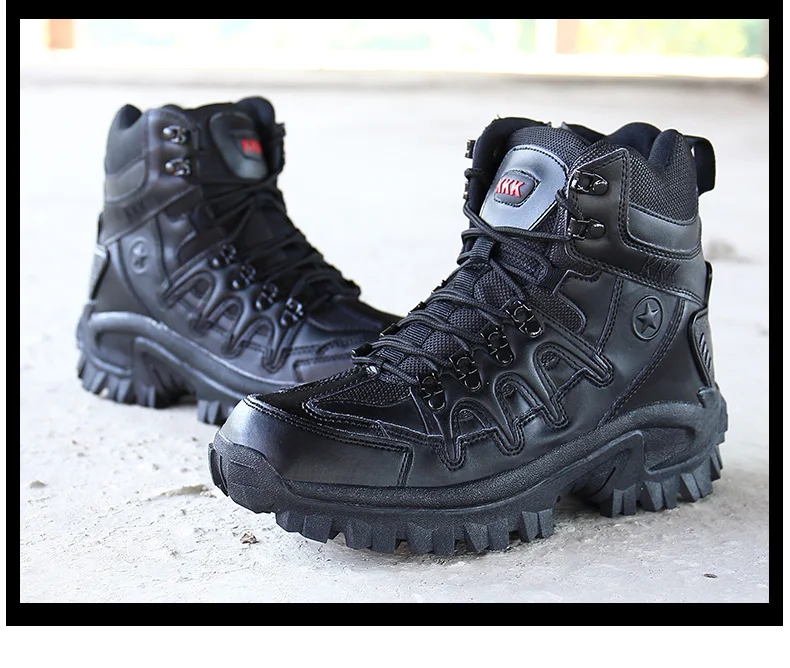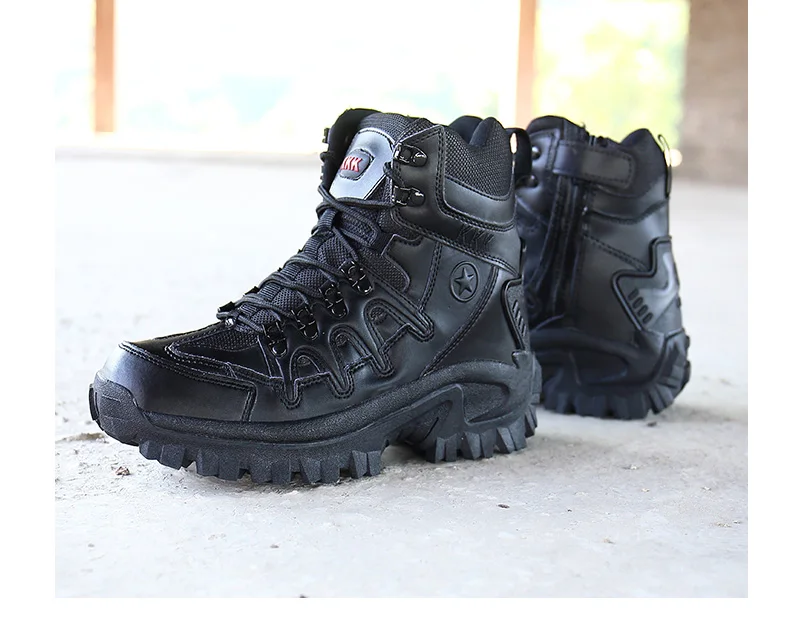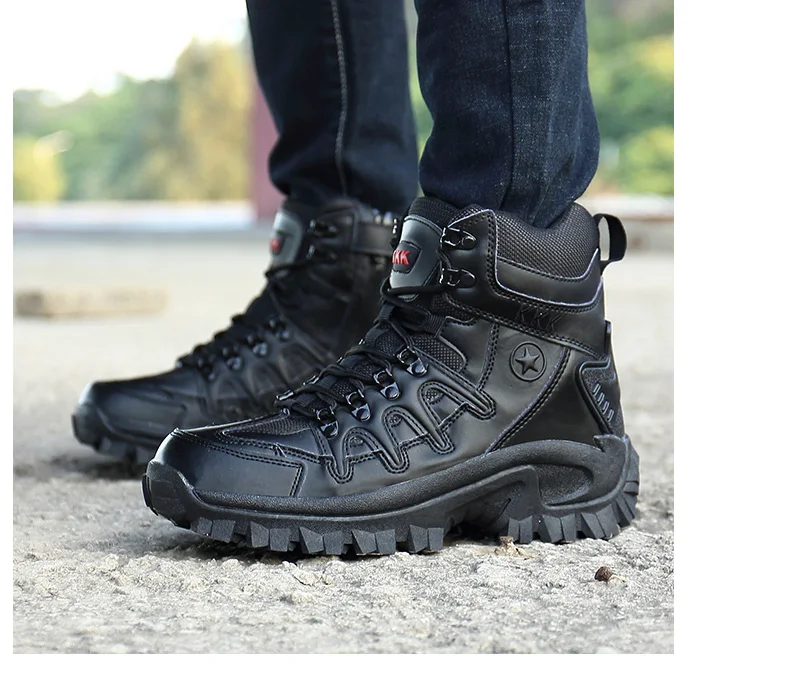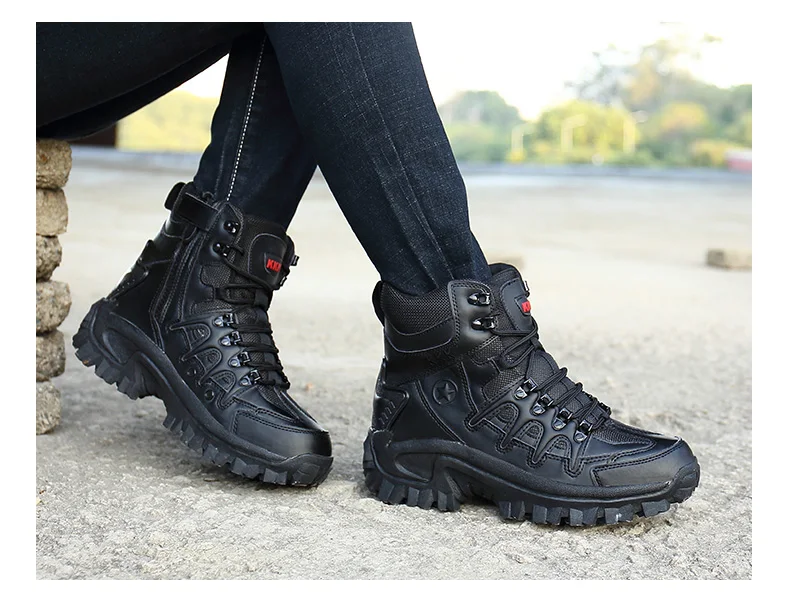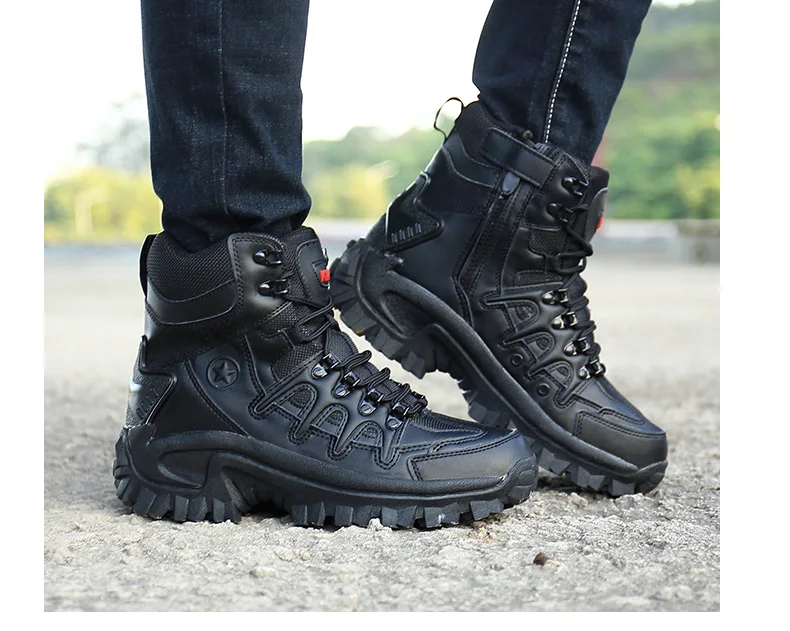# Us Army Boot Combat Mens Ankle Boot Tactical Big SizeImportant Notice:
Foot length is not the insole length.
Foot length is not the outsole length.
Foot length < Insole Length < Outsole Length

***Size chart***
EU Size38 = US6 =Foot Length 24.0cm
EU Size39 =US6.5 =Foot Length 24.5cm
EU Size40 =US7 =Foot Length 25.0cm
EU Size41 =US8 =Foot Length 25.5cm
EU Size42 =US8.5 =Foot Length 26.0cm
EU Size43 =US9.5 =Foot Length 26.5cm
EU Size44 =US10 =Foot Length 27.0cm
EU Size45 =US11 =Foot Length 27.5cm
EU Size46 =US12 =Foot Length 28.0cm
EU Size47 =US12.5 =Foot Length 28.5cm